# Crash Course in Machine Learning

## A survey of machine learning algorithms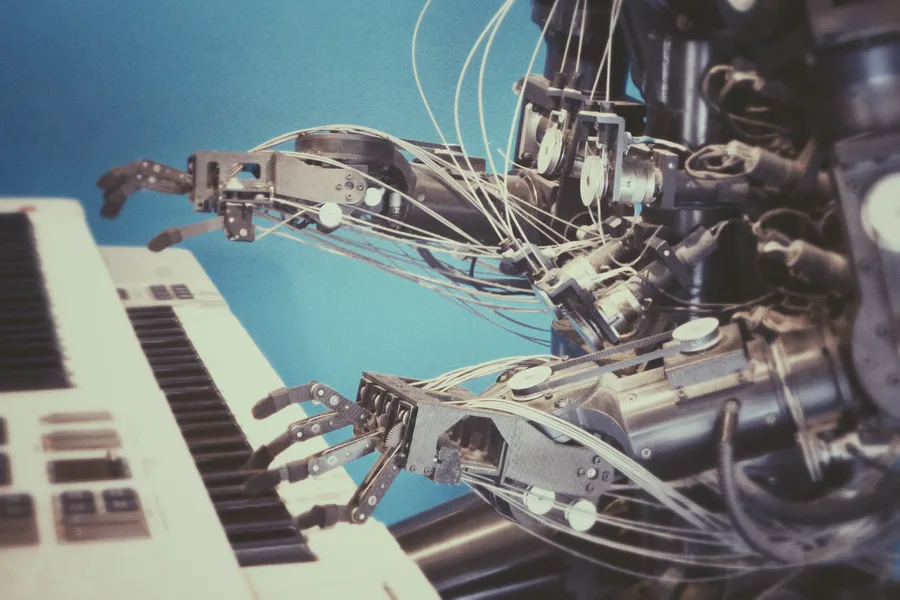Rafael Ballestas

November 19, 2018

In this article we clarify some of the undefined terms in our previous article and thereby explore a selection of machine learning algorithms and their applications to information security.

This is not meant to be an exhaustive list of all machine learning (`ML`) algorithms and techniques. We would like, however, to demistify some obscure concepts and dethrone a few buzzwords. Hence, this article is far from neutral.

First of all we can classify by the type of input available. If we are trying to develop a system that can identify if the animal in a picture is a cat or a dog, initially we need to train it with pictures of cats or dogs. Now, do we tell the system what each picture contains? If we do, it’s supervised learning and we say we are training the system with labeled data. The opposite is completely unsupervised learning, with a few levels in between, such as

• semi-supervised learning: with partially labeled data

• active learning: the computer has to "pay" for the labels

• reinforcement learning: labels are given as output starts to be produced

However, each algorithm typically fits more naturally either in the supervised or unsupervised learning category, so we will stick to those two.

Next, what do want to obtain from our learned sytem? The cat-dog situation above is a typical classification problem; given a new picture, to what category does it belong? A related but different problem is that of clustering, which tries to divide the inputs into groups according to the features identified in them. The difference is that the groups are not known beforehand, so this is an unsupervised problem.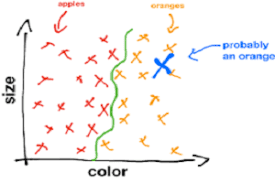Figure 1. Classification.

Both of these problems are discrete, in the sense that categories are separate and there are no in-between elements (a picture that is 80% cat and 20% dog). What if my data is continuous? Good old regression to the rescue! Even the humble least squares linear regression we learned in school can be thought of as a machine learning technique, since it learns a couple of parameters from the data and can make predictions based on those.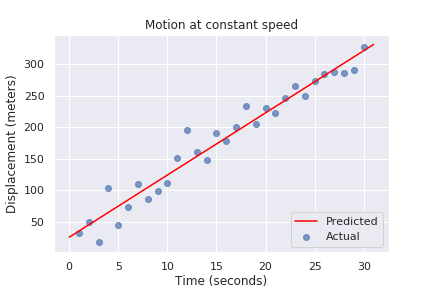Figure 2. Linear Regression

Two other interesting problems are estimating the probability distribution of a sample of points (density estimation) and finding a lower-dimensional representation of our inputs (dimensionality reduction):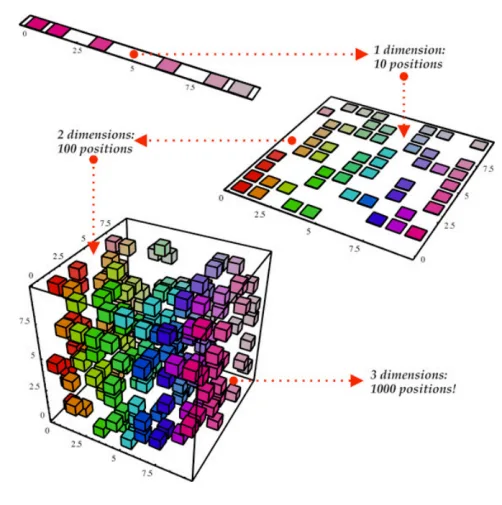Figure 3. Dimensionality reduction.

With these classifications out of the way, let’s go deeper into each particular technique that is interesting for our purposes.

## Support vector machines

Much like linear regression tries to draw a line that best joins a set of closely correlated points, support vector machines (`SVM`) try to draw a line that separates a set of naturally separated points. Since a line divides the plane in two, any new point must be on one of the two sides, and is thus classified as belonging to one class or the other.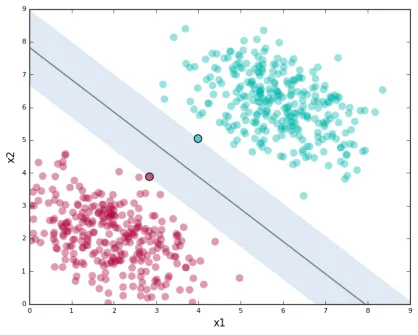Figure 4. Support Vector Machines in `2D` and `3D`.

More generally, if the inputs are n-dimensional vectors, an `SVM` tries to find a geometric object of dimension n-1 (a hyperplane) that divides the given inputs into two groups. To name an application, support vector machines are used to detect spam in images (which is supposed to evade text spam filters) and face detection.

## K-means clustering

We need to group unlabeled data in a meaningful way. Of course, the number of possible clusterings is very large. In the k-means technique, we need to specify the desired number of clusters k beforehand. How do we choose? We need a way to measure cluster compactness. For every cluster we can define its centroid, something like its center of mass. Thus a measure of the compactness of a cluster could be the sum of the member-to-centroid distances, called the distortion: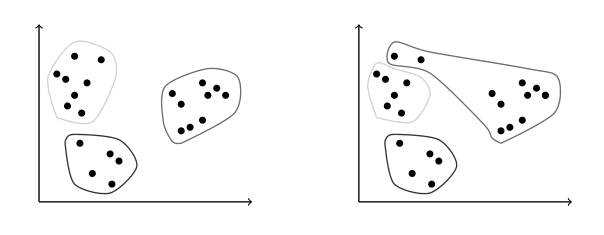Figure 5. Distortion is lower on the left than on the right, so compactness is better.

With that defined, we can state the problem clearly as an optimization problem: minimize the sum of all distortions. However, this problem is `NP`-complete (computationally very difficult) but good estimations can be achieved via k-means. It can be shown and, more importantly, makes intuitive sense, that:

1. Each point must be clustered with the nearest centroid.

2. Each centroid is at the center of its cluster.

Clustering has been used in the context of security for malware detection; see for example Pai (2015) and Pai et al. (2017).

## Artificial neural networks and deep learning

Loosely inspired by the massive parallelism animal brains are capable of, these models are highly interconnected graphs in which the nodes are (mathematical) functions and the edges have weights, which are to be adjusted by the training. A set of weights is scored by the accuracy of labeled output, and optimized in the next step or epoch of training in a process called back-propagation (of error). The weights are adjusted in such a way that the measured error decreases. The nodes are arranged in layers and their functions are typically smooth versions of step functions (i.e., yes/no functions, but with no big jumps), and there are two special layers for input and output. After training, since the whole network is fixed, it’s only a matter of giving it input and getting the output.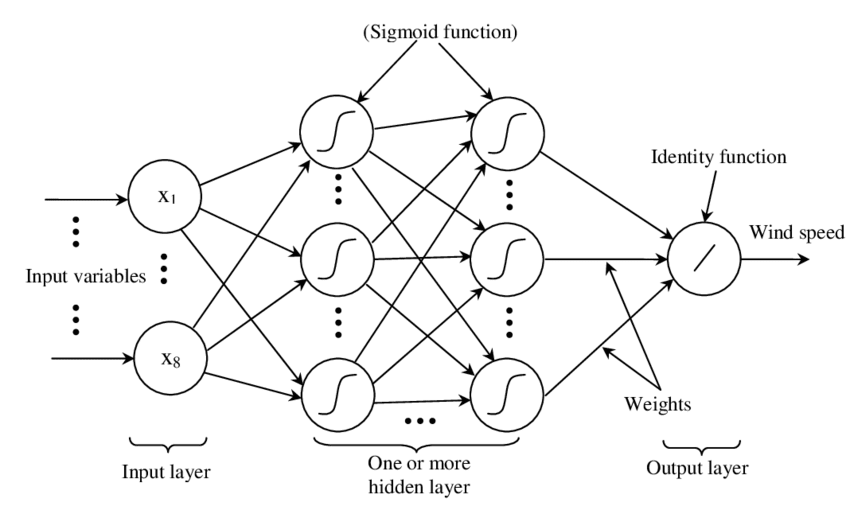Figure 6. A neural network with two layers.

The networks described above are feed-forward, but there are also recurrent neural networks. Convolutional networks use mathematical cross-correlation instead of regular smooth step functions. Deep neural networks owe their name to the great number of layers they use and to the fact that they are unsupervised learning models.

While these networks have been quite succesful in applications, they are not perfect:

• in contrast to simpler machine learning models, they don’t produce an understandable model; it’s just a black box that computes output given input.

• biology is not necessarily the best model for engineering. In Mark Stamp’s words ,

Attempting to construct intelligent systems by modeling neural interactions within the brain might one day be seen as akin to trying to build an airplane that flaps its wings.

## Decision trees and forests

In stark contrast to the unintelligible models extracted from neural networks, decision trees are simple enough to understand at a glance: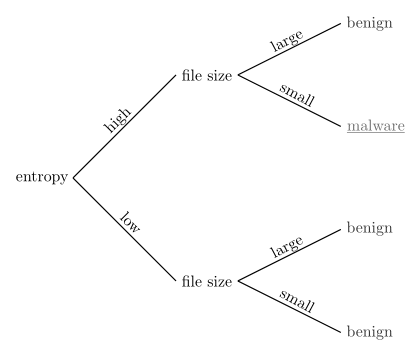Figure 7. A decision tree for classifying malware. Taken from .

However, decision trees have a tendency to overfit the training data, i.e., are sensitive to noise and extreme values in it. Worse, a particular testing point could be predicted differently by two trees made with the same training data, but with, for example, the order of features reversed.

These difficulties can be overcome by constructing many trees with different (even possibly overlapping) subsets of the training data and making the final conclusion by choosing from among all the trees' decisions. This solves overfitting, but the intution obtained from simple trees is lost.

## Anomaly detection via k-nearest neighbors

Detecting anomalies is a naturallyunsupervised problem and really makes up a whole class of algorithms and techniques, more data mining than machine learning.

The k-nearest neighbors algorithm (`kNN`), essentially classifies an element according to the k training elements closest to it.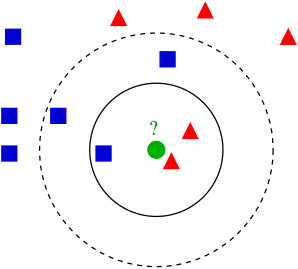Figure 8. The new point would be classified as a triangle in `3NN`, but as a square in `5NN`.

The `kNN` algorithm can also be adapted to be used in the context of regression, classification, and anomaly detection, in particular by scoring elements in terms of the distance to its closest neighbor (`1NN`).

Notice that in `kNN` there is no training phase. the labeled input is the training data and the model in itself. The most natural application for anomaly detection in computer security is in intrusion detection systems.

I hope this article has served to establish the following general ideas on machine learning:

• Even though `ML` has gained a lot of momentum in the past few years, its basic ideas are quite old.

• Fancy names can sometimes be used to masquerade simple ideas.

• `ML` is not a field of its own, rather an application of statistics, optimization, data analysis and data mining.

## References

1. Mark Stamp (2018). Introduction to Machine Learning with Applications in Information Security. CRC Press.

Share

Tags:

## Recommended blog posts

You might be interested in the following related posts.Get an overview of vulnerability assessmentBenefits of continuous over point-in-time pentestingFor which security standards is pentesting a must-have?Pentesting is a system-agnostic approach to securityInjecting JS into one site is harmful, into all, lethalDifferences between these security testing approachesOur CLI is an approved AST tool to secure cloud appsHow BAS solutions work, their importance and benefits

## Start your 21-day free trial

Discover benefits of our Continuous Hacking solution, which hundreds of organizations are already enjoying.